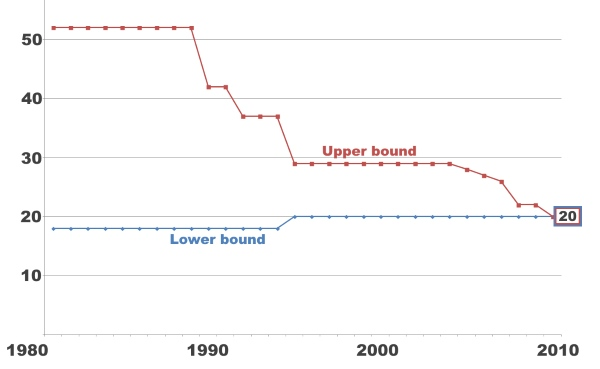# What is Rubik’s God number?

Last Updated on April 13, 2023 by Francis

Are you familiar with the Rubik’s Cube? It is one of the world’s most popular puzzles, with more than 350 million cubes sold worldwide. But did you know that there is a certain “God number” associated with the cube? This number is the minimum number of moves it takes to solve the cube. In this article, we will discuss what this “God number” is and why it is so important. So, get ready to explore the mysterious world of the Rubik’s Cube and its “God number”!Contents

## What is the God Number of the Rubik’s Cube?

The Rubik’s Cube is a 3D puzzle game invented by Hungarian sculptor and professor of architecture Ernö Rubik in 1974. The cube has six sides, each containing nine colored squares, and each side can be rotated independently. The goal of the game is to rotate the sides of the cube so that all of the colored squares on each side are in the same order. To do this, the player must use a series of algorithms and moves to solve the puzzle. The God Number of the Rubik’s Cube is the fewest number of moves needed to solve the cube from any starting position.

### The God Number of the Rubik’s Cube

The God Number of the Rubik’s Cube is 20. This means that any Rubik’s Cube can be solved in 20 moves or less. In August 2010, a computer program developed by Google engineer and mathematician Morley Davidson was able to solve the Rubik’s Cube in the fewest number of moves, 20. This was the first time that anyone had solved the puzzle in the fewest number of moves, and the program was named “God’s Number” in honor of this achievement.

### What Makes the Rubik’s Cube Difficult?

The Rubik’s Cube is a difficult puzzle to solve because of its many permutations. There are over 43 quintillion (43,252,003,274,489,856,000) possible permutations of the cube, which makes it difficult to solve. Each move of the cube changes its configuration, and it can take a lot of trial and error to find the correct solution.

### Solving the Rubik’s Cube

The most common way to solve the Rubik’s Cube is to use an algorithm. Algorithms are a set of steps that are used to solve a problem. There are many different algorithms that can be used to solve the Rubik’s Cube. Some of the most popular algorithms include the Fridrich Method, the CFOP Method, the Yau Method, and the Ortega Method.

### Tips for Solving the Rubik’s Cube

The best way to learn how to solve the Rubik’s Cube is to practice. You can also try watching tutorials online or reading books about solving the cube. It is important to remember to take your time and think carefully before making each move. Additionally, you should try to break down the cube into smaller parts and solve each part one at a time.

### Rubik’s Cube Competitions

Rubik’s Cube competitions are held all over the world. These competitions are divided into two categories: speedcubing and blindfolded cubing. Speedcubing is a race to see who can solve the cube the fastest, while blindfolded cubing is a race to see who can solve the cube without being able to see it. Competitors in these competitions must adhere to a strict set of rules and regulations.

### The World Cube Association

The World Cube Association (WCA) is the governing body for Rubik’s Cube competitions. The WCA was founded in 2004 and is responsible for organizing and running competitions around the world. The WCA also maintains the official world rankings for Rubik’s Cube solvers and publishes information about upcoming competitions.

### What is Rubik’s God Number?

Answer: Rubik’s God Number is the minimum number of moves needed to solve any given Rubik’s Cube. It is also known as the “half turn metric” or the “God’s number”. The God Number for the 3x3x3 Rubik’s Cube is 20, meaning any combination of the cube can be solved in at most 20 moves.

### What is the significance of Rubik’s God Number?

Answer: Rubik’s God Number is significant because it represents the upper limit of how many moves it takes to solve any given Rubik’s Cube. Knowing this upper limit allows for efficient algorithms to be created for solving the cube in the least amount of moves. It also helps to determine the difficulty of a Rubik’s Cube, with higher numbers indicating a more difficult puzzle.

### How was Rubik’s God Number determined?

Answer: Rubik’s God Number was determined by a series of exhaustive computer searches conducted by computer scientists and mathematicians at the University of Exeter in the early 2000s. They used a technique called “group theory” to determine the number of moves needed to solve any given Rubik’s Cube, and eventually arrived at the answer of 20.

### Are there other Rubik’s Cubes with different God Numbers?

Answer: Yes, there are other Rubik’s Cubes with different God Numbers. For example, the 2x2x2 Rubik’s Cube has a God Number of 11, while the 4x4x4 Rubik’s Cube has a God Number of 50. The higher the God Number, the more difficult the Rubik’s Cube is to solve.